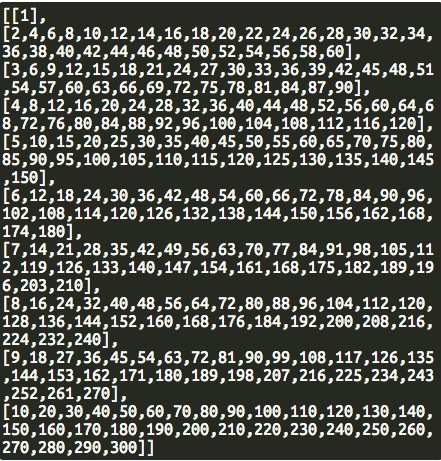# Why is this array not pushing right numbers to first element?

Here is the full code:

``````Array.prototype.allValuesSame = function() {

for(var i = 1; i < this.length; i++)
{
if(this[i] !== this)
return false;
}

return true;
};

function smallestCommons(arr) {

var firstNum = arr;
var secondNum = arr;
var array = [];

if(secondNum>firstNum){
for(var i = firstNum; i <= secondNum; i++){
array.push([i]);
}
}else if(secondNum<firstNum){
for(var j = secondNum; j <= firstNum; j++){
array.push([j]);
}
}

var test = [];
for(var z=0; z<array.length; z++){
test.push([]);
}

for(var t=0, x=1; t<array.length; t++){
test[t].push(array[t]*x);
if(t == array.length-1){
t=0;
x+=1;
}
if(x>30){
break;
}
}

return (test);
}
``````

The loop that is supposed to push the multiples to the array is here:
` for(var t=0, x=1; t<array.length; t++){ test[t].push(array[t]*x); if(t == array.length-1){ t=0; x+=1; } if(x>30){ break; } }`As you can see, it doesnt push all the multiples of 1 to the first array. If anyone knows how to fix it that would be great!

Hey,

it does not push the multiples of 1 to the first array because “t” will never be 0 again.

``````for(var t=0, x=1; t<array.length; t++){
test[t].push(array[t]*x);
if(t == array.length-1){
t=0;
x+=1;
}
if(x>30){
break;
}
}
``````

Your first if-statemant will set t to 0 if you reach your last Array (in your case the 10th array).
But after this if-statement (and the second one) is completed your for-loop will start a new iteration. On each iteration your loop increments t by 1 (because of t++).
This means t - which was previously set to “0” - will now be incremented to be"1".

Setting t inside your if-statement to be “-1” sould do the trick.

1 Like

I think if you want to solve this with less code and loops, you should study up on the euclidean algorithm.
Basically lcm(a, b) = gcd(a, b)/a * b

If you research on finding the gcd finding lcm will be easier.

1 Like

ok thank you, will keep that in mind

Hello I recently joined FCC so I am new to all this, but I have learnt programming before so I might be able to help.
What exactly is the program trying to accomplish?

This is for the Smallest Common Multiple Algorithm Challenge, found here: https://www.freecodecamp.com/challenges/smallest-common-multiple

That for loop in particular was multiplying each number in the array by x, and pushin x into an array.

I will just write the logic you have to use, as I am sure you will be able to code it.
So the problem asks to find the smallest common multiple (or LCM) of two numbers which are also divisible by all numbers between the two numbers.
one way to do it is to find the bigger of the two numbers, and find a number that is divisible by the bigger number and (bigger number)-1 (call it x) (it should always be (bigger number)(x) since they are both relative primes). call this answer as y
next you decrement x, and find out if x (bigger number-2) is divisible by y. If it is then decrement x and check if it is divisible by y. if you reach a point where x isint divisible, then y =y
x, and continue the decrementing.
Do this until x ==smaller number
I hope you are able to understand this, as I am not the best in explaining logic.
If you still do not get it, I wouldn’t mind giving you an example and also showing the code in C++, as I do not know the JavaScript syntax yet(both are very similar though).